## Discriminant (Polynomial)

The Product of the Squares of the differences of the Polynomial Roots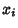. For a Polynomial of degree,(1)

The discriminant of the Quadratic Equation(2)

is usually taken as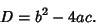(3)

However, using the general definition of the Polynomial Discriminant gives(4)

whereare the Roots.

The discriminant of the Cubic Equation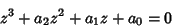(5)

is commonly defined as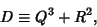(6)

where(7)(8)

However, using the general definition of the polynomial discriminant for the standard form Cubic Equation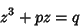(9)

gives(10)

whereare the Roots and(11)

The discriminant of a Quartic Equation(12)

is(13)
(Beeler et al. 1972, Item 4).